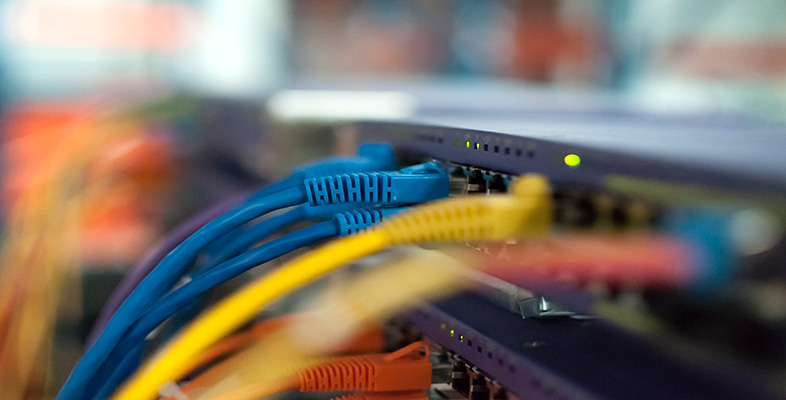Science, Maths & Technology

### Become an OU studentIT: device to device communication

Start this free course now. Just create an account and sign in. Enrol and complete the course for a free statement of participation or digital badge if available.

# 2.7 Propagation delay

The time taken for a signal to travel from its source to its destination is known as propagation delay. This is derived from the verb 'propagate' which in a physics context means 'spread' or 'travel'. The propagation delay depends on a number of factors, including the distance the signal has to travel and the signal's speed.

Contemporary physics states that nothing can travel faster than the speed of light (or any electromagnetic wave) in a vacuum which, to the nearest metre, is 299 792 458 metres per second. This is a rather precise measurement and for most purposes we don't need to work to this degree of accuracy. You are more likely to see the speed of light given as 'approximately 3×108 m/s' (that is 300 000 000 metres per second). It's important to realise that not all carriers are able to achieve the speed of light. A voltage pulse travelling in a copper cable has a speed of approximately 2×108 m/s. Signals can also be delayed by the processes required to manipulate and manage them on their journey from sender to receiver.

It is often acceptable to round numbers up or down to make calculations simpler. The result won't be completely precise, but it will probably be close enough for many purposes. The box below takes the idea further and explains the principles.

## Rounding numbers

The more digits there are in a number, the less contribution the digits at the right-hand end make to the magnitude of the number. Consider Table 2. Incrementing 9 by 1 results in an increase of the original number by almost one-tenth. Incrementing 99 by 1 results in an increase of the original number by almost one-hundredth, and so on. The longer the number, the less is the effect of an increase in the right-most digit. Or, put another way, the longer the number, the less significant the right-most digit becomes.

Table 2: Fractional comparisons
Original number New number Fractional increase Approximate fraction of original number
9101/9one-tenth
991001/99one-hundredth
9991 0001/999one-thousandth
9 99910 0001/9 999one ten-thousandth
99 999100 0001/99 999one hundred-thousandth
999 9991 000 0001/999 999one-millionth

This means that in long numbers we can often disregard some of the digits at the right-hand end because they are not significant. Disregarding them will introduce very little error because they make very little contribution to the overall value. It is the digits at the left-hand end that are significant, and we can select the degree of accuracy required by choosing how many left-hand digits we consider to be significant for our purposes. We call these significant figures. Then we round the original number up or down until all but the required significant figures are shown as 0s.

For example, take the number 3482. Let's say we decide that we need to work with 3 significant figures. In this case we would round the original number down to 3480 because 3482 is closer to 3480 than it is to 3490.

Let's say instead that we decide to work with 2 significant figures. In this case I would round 3482 up to 3500 because 3482 is closer to 3500 than it is to 3400.

When we round a number up or down in this way it is important to indicate what we have done by stating that the rounded number is approximate, or by stating how many significant figures we are working to. Thus 3482 becomes:

3480 (correct to 3 significant figures)

or

3500 (correct to 2 significant figures)

These rules apply just the same when we are working with long numbers that include a decimal point, or with long numbers expressed in scientific notation. For example 2.14565879×108 would become 2.146×108 (correct to 4 significant figures), or 2.15×108 (correct to 3 significant figures).

The speed of light in a vacuum (299 792 458 m/s) gives a good example of why it can sometimes be useful to express how many significant figures we are working to. The figure becomes:

299 800 000 (correct to 4 significant figures)

and

300 000 000 (correct to 3 significant figures)

because the rounding (up in this case) gives a result that might otherwise be taken to be correct to only 1 significant figure.